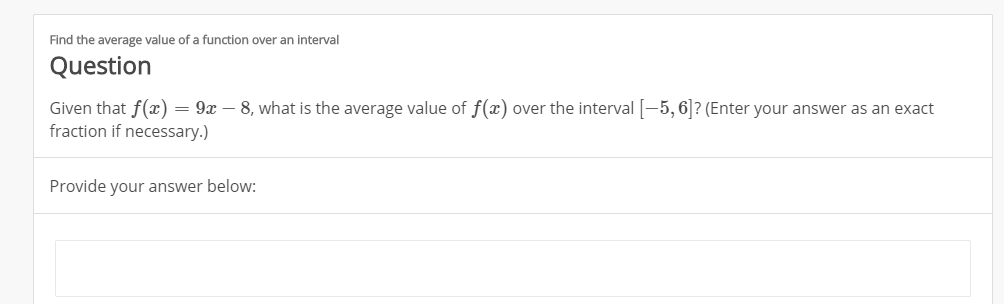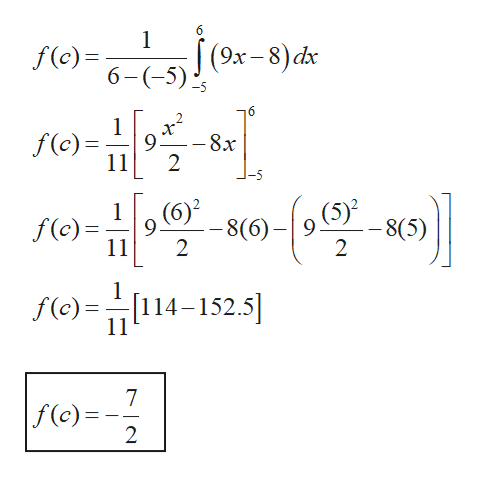# Find the average value of a function over an intervalQuestionGiven that f(xfraction if necessary.)= 9x 8, what is the average value of f(x) over the interval-5,6? (Enter your answer as an exactProvide your answer below:

Question
1 viewshelp_outlineImage TranscriptioncloseFind the average value of a function over an interval Question Given that f(x fraction if necessary.) = 9x 8, what is the average value of f(x) over the interval-5,6? (Enter your answer as an exact Provide your answer below: fullscreen
check_circle

star
star
star
star
star
1 Rating
Step 1

To find the average value, we integrate the function over the given interval and divide it by the total interval.

Step 2

Consider the given function and the i...help_outlineImage Transcriptionclose6 1 f(6--5) (9x-8)dx -5 6 f(c)= 11 -8x 2 (5)2 1 (6)2 9 f(c) -8(6) - 8(5) 9 11 2 2 f(e)=114-152.5 11 7 f(c)=- 2 fullscreen

### Want to see the full answer?

See Solution

#### Want to see this answer and more?

Solutions are written by subject experts who are available 24/7. Questions are typically answered within 1 hour.*

See Solution
*Response times may vary by subject and question.
Tagged in

### Integration